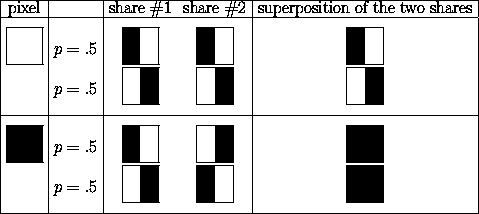# Doug Stinson's Visual Cryptography Page

Visual cryptography was introduced by Naor and Shamir at EUROCRYPT '94. They asked the following intriguing question: is it possible to devise a secret sharing scheme in which an image can reconstructed "visually" by superimposing two shares? Each share would consist of a transparency, made up of black and white pixels. (Note that it would be more accurate to say "transparent" rather than "white".) Examination of one share should reveal no information about the image.

Naor and Shamir devised the following scheme, illustrated in the figure below. The algorithm specifies how to encode a single pixel, and it would be applied for every pixel in the image to be shared.A pixel P is split into two subpixels in each of the two shares. If P is white, then a coin toss is used to randomly choose one of the first two rows in the figure above. If P is black, then a coin toss is used to randomly choose one of the last two rows in the figure above. Then the pixel P is encrypted as two subpixels in each of the two shares, as determined by the chosen row in the figure. Every pixel is encrypted using a new coin toss.

Suppose we look at a pixel P in the first share. One of the two subpixels in P is black and the other is white. Moreover, each of the two possibilities "black-white" and "white-black" is equally likely to occur, independent of whether the corresponding pixel in the secret image is black or white. Thus the first share gives no clue as to whether the pixel is black or white. The same argument applies to the second share. Since all the pixels in the secret image were encrypted using independent random coin flips, there is no information to be gained by looking at any group of pixels on a share, either. This demonstrates the security of the scheme.

Now let's consider what happens when we superimpose the two shares (here we refer to the last column of the figure). Consider one pixel P in the image. If P is black, then we get two black subpixels when we superimpose the two shares; if P is white, then we get one black subpixel and one white subpixel when we superimpose the two shares. Thus, we could say that the reconstructed pixel (consisting of two subpixels) has a grey level of 1 if P is black, and a grey level of 1/2 if P is white. There will be a 50% loss of contrast in the reconstructed image, but it should still be visible.

Since the summer of 1995, I have been involved in research on this topic, partly in collaboration with C. Blundo and A. De Santis of the University of Salerno. Here are a few papers of mine on this subject, available as postscript files:

I published an introductory article on visual cryptography in the April 1998 issue of Dr. Dobb's Journal.

I have given seminar talks on this topic many times in recent years. The slides for the most recent version of the talk are available here, in the form of a postscript file. The talk is entitled "Visual Cryptography: Seeing is Believing".

Some examples of visual cryptography are provided.

• A two out of two threshold scheme. The image is the the Canadian flag
• A two out of two threshold scheme. The image is the the Greek letter "pi"
• A scheme for a graph access structure. In this scheme, the image will be visible if shares #1 and #2 are superimposed; or if shares #2 and #3 are superimposed; or if shares #3 and #4 are superimposed. The image will not be visible if shares #1 and #3 are superimposed; or if shares #1 and #4 are superimposed; or if shares #2 and #4 are superimposed. The image is a happy face

Further information and examples can be found at the University of Salerno web page on visual cryptogrpahy.